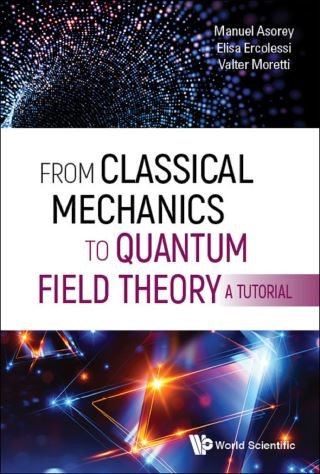## From Classical Mechanics to Quantum Field Theory, A Tutorial• Description
• Author
• Info
• Reviews

### Description

This book collects an extended version of the lectures delivered by the authors at the Fall Workshop on Geometry and Physics in the years 2014, 2015, 2016.

It aims at introducing advanced graduate and PhD students, as well as young researchers, to current research in mathematics and physics. In particular, it fills the gap between the more physical-oriented and the more mathematical-oriented literature on quantum theory. It introduces various approaches to methods of quantization, along with their impact on modern mathematical methods.

Contents:
• A Short Course on Quantum Mechanics and Methods of Quantization:
• Introduction
• Overview of Quantum Mechanics
• Methods of Quantization
• Mathematical Foundations of Quantum Mechanics: An Advanced Short Course:
• Introduction: Summary of Elementary Facts of QM
• Observables in Infinite Dimensional Hilbert Spaces: Spectral Theory
• More Fundamental Quantum Structures
• Just Few Words about the Algebraic Approach
• A Concise Introduction to Quantum Field Theory:
• Introduction
• Quantum Mechanics and Relativity
• Quantum Field Theory
• The Quantum Vacuum
• Fields versus Particles
• Fields in Interaction
• Covariant Approach
• Conformal Invariant Theories
• What is Beyond?
• Appendix 1. Casimir Effect
• Appendix 2. Gaussian Measures
• Appendix 3. Peierls Brackets

Readership: Advanced graduate students, PhD students and young researchers, both in mathematics and physics, interested in Classical Mechanics and Quantum Field Theory.Classical Mechanics;Quantum Mechanics;Methods of Quantization;Geometric Quantum Mechanics;Algebraic Formulation of Quantum Mechanics;Quantum Field Theory0Key Features:
• It fills the gap between the mathematical-oriented and the physical-oriented literature on the subject
• It presents in a unique volume the different approaches to quantization, starting from the ideas of classical mechanics up to the development of quantum field theories
• It introduces the reader to current research topics in a pedagogical way